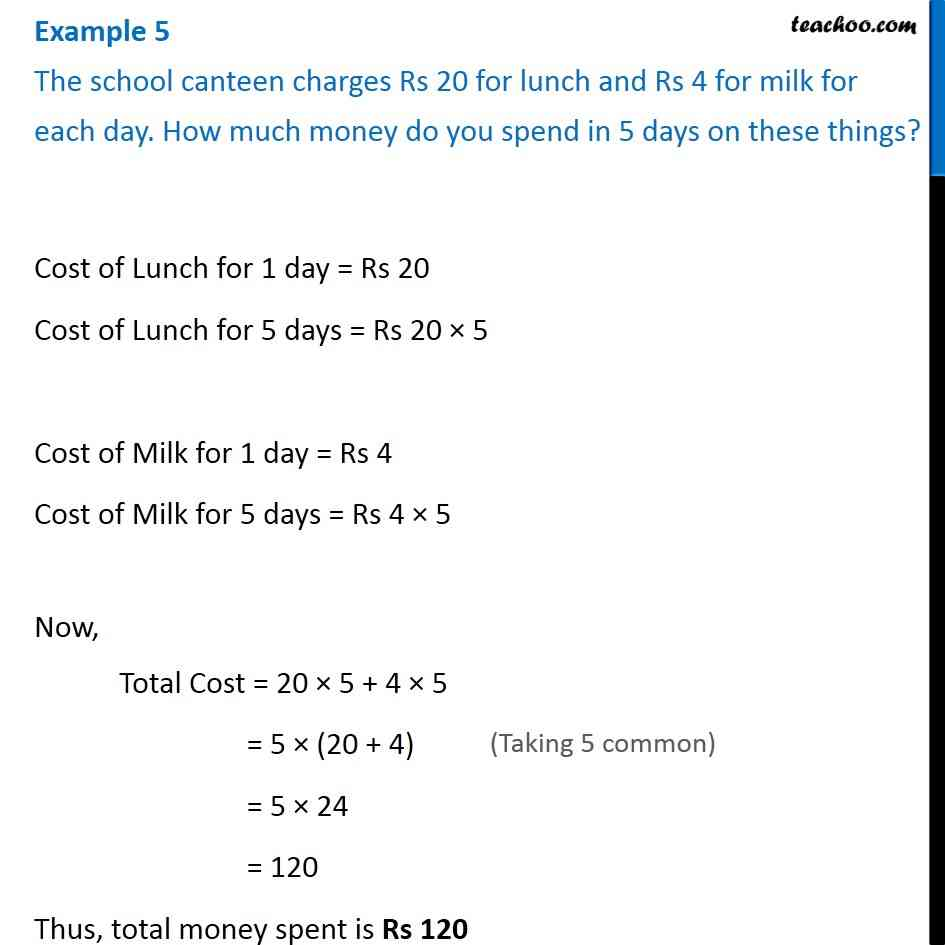Practice Questions

Chapter 2 Class 6 Whole Numbers
Serial order wiseLearn in your speed, with individual attention - Teachoo Maths 1-on-1 Class

### Transcript

Question 5 - Chapter 2 Class 6 Whole Numbers - NCERT Book The school canteen charges Rs 20 for lunch and Rs 4 for milk for each day. How much money do you spend in 5 days on these things? Cost of Lunch for 1 day = Rs 20 Cost of Lunch for 5 days = Rs 20 × 5 Cost of Milk for 1 day = Rs 4 Cost of Milk for 5 days = Rs 4 × 5 Now, Total Cost = 20 × 5 + 4 × 5 = 5 × (20 + 4) = 5 × 24 = 120 Thus, total money spent is Rs 120